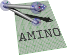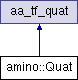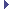amino  1.0-beta2 Lightweight Robot Utility Library
amino::Quat Struct Reference

A quaternion object. More...

#include <tf.hpp>

Inheritance diagram for amino::Quat:## Public Member Functions

Quat ()
Construct an identity quaternion.

Quat (const aa_tf_quat *p)
Construct from another quaternion.

Quat (const aa_tf_quat &p)
Construct from another quaternion.

Quat (const aa_tf_rotmat *p)
Construct from a rotation matrix.

Quat (const aa_tf_rotmat &p)
Construct from a rotation matrix.

Quat (const aa_tf_axang *p)
Construct from an axix angle rotation.

Quat (const aa_tf_axang &p)
Construct from an axix angle rotation.

Quat (const XAngle &p)
Construct from a rotation about the x axis.

Quat (const YAngle &p)
Construct from a rotation about the Y axis.

Quat (const ZAngle &p)
Construct from a rotation about the Z axis.

## Static Public Member Functions

static aa_tf_quat from_quat (const double x)
Create a quaternion object from a quaternion array.

static aa_tf_quat from_xyzw (double x, double y, double z, double w)
Create a quaternion object from components.

static aa_tf_quat from_rotmat (const double x)
Create a quaternion object a rotation matrix.

static aa_tf_quat from_axang (const double x)
Create a quaternion object an axis-angle.

static aa_tf_quat from_axang (const double a, double angle)
Create a quaternion object an axis-angle.

static aa_tf_quat from_rotvec (const double x)
Create a quaternion object a rotation vector.

static aa_tf_quat from_xangle (const double v)
Create a quaternion object rotation about x.

static aa_tf_quat from_yangle (const double v)
Create a quaternion object rotation about y.

static aa_tf_quat from_zangle (const double v)
Create a quaternion object rotation about z.Public Attributes inherited from aa_tf_quat
union {
struct {
double   x
x component

double   y
y component

double   z
z component

double   w
w component

}

struct {
union {
struct aa_tf_vec3   vec
vector part

double   v 
vector part

}

double   scalar
scalar part

}

double   data 
data array

};

## Detailed Description

A quaternion object.

Definition at line 174 of file tf.hpp.

The documentation for this struct was generated from the following file: